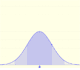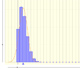Normal distribution: Symmetric intervals

Using the (cumulative) distribution function we can calculate the probability of any interval:The (cumulative) distribution function of a random variable X, evaluated at x, is the probability that X will take a value less than or equal to x. In this page we study the Normal Distribution.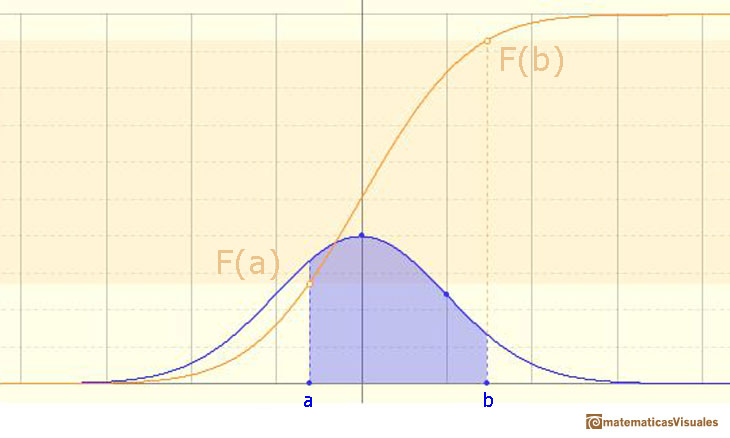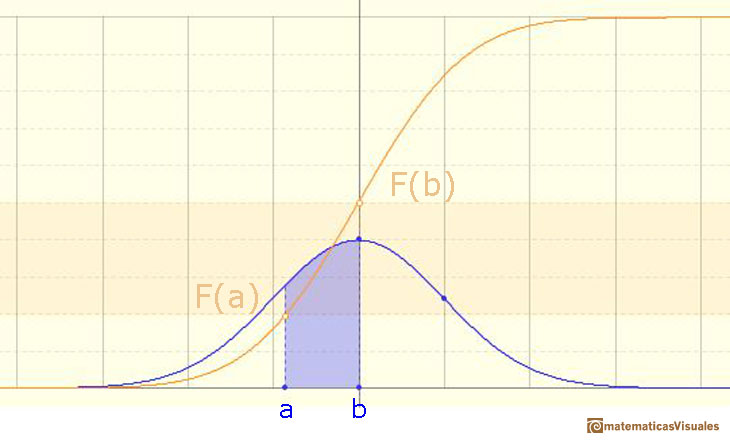In this page we are going to study one simple and interesting case: symmetric intervals around the mean.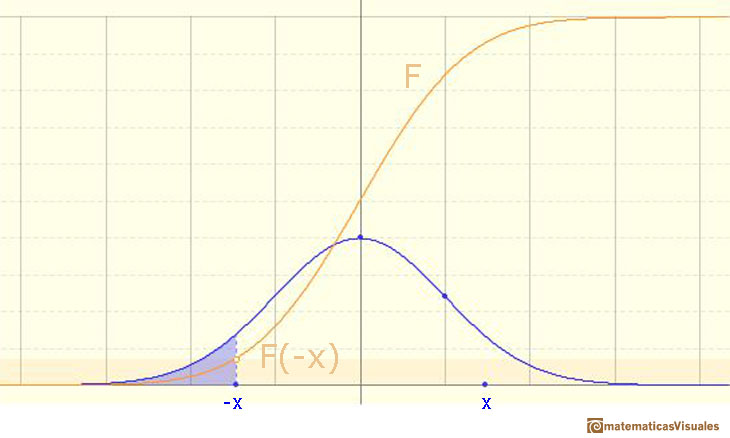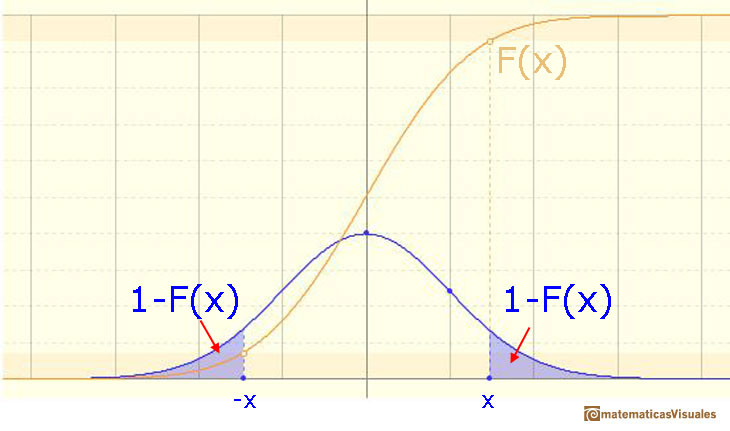Then

We can modifying the parameters of the normal distribution.

The mean is represented by a triangle that can be seen as an equilibrium point. By dragging it we can modify the mean.

Dragging the point on the curve (which is one of the two inflexion points of the curve) we modify the standard deviation.

Moving the blue point on the X-axis we can change the symmetric interval.

We can see the cumulative distribution function and how it change by modifiyng the mean (simple translation) and the standard deviation (reflecting greater or lesser dispersion of the variable).

The red dots control the vertical and horizontal scales of the graphic.

REFERENCES

George Marsaglia's article Evaluating the Normal Distribution.
Saul Stahl's article The Evolution of the Normal Distribution (Mathematics Magazine, vol. 79, (2006), pp. 96-113. From Mathematical Association of America).